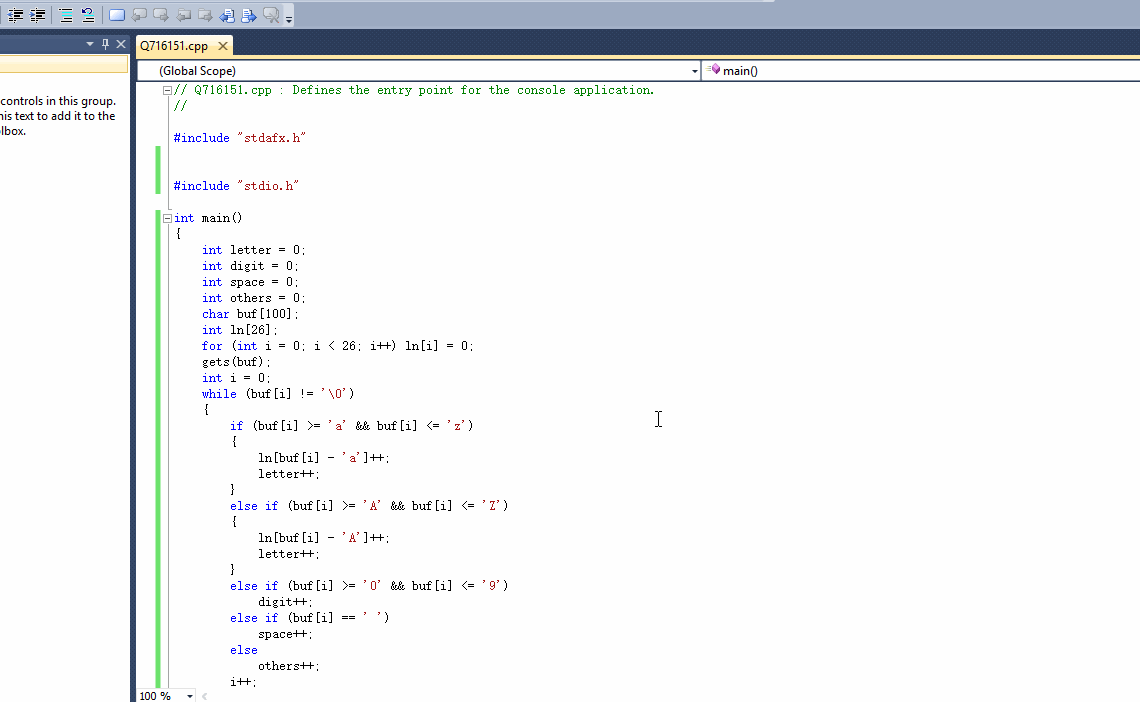C语言：统计字符串中字符的种类

0

2个回答

# 如果问题得到解决，请点我回答左上角的采纳和向上的箭头，谢谢

``````#include "stdio.h"

int main()
{
int letter = 0;
int digit = 0;
int space = 0;
int others = 0;
char buf;
int ln;
for (int i = 0; i < 26; i++) ln[i] = 0;
gets(buf);
int i = 0;
while (buf[i] != '\0')
{
if (buf[i] >= 'a' && buf[i] <= 'z')
{
ln[buf[i] - 'a']++;
letter++;
}
else if (buf[i] >= 'A' && buf[i] <= 'Z')
{
ln[buf[i] - 'A']++;
letter++;
}
else if (buf[i] >= '0' && buf[i] <= '9')
digit++;
else if (buf[i] == ' ')
space++;
else
others++;
i++;
}
int total = i - 1;
printf("L=%d D=%d S=%d O=%d T=%d\n", letter, digit, space, others, total);
for (int i = 0; i < 26; i++)
{
if (ln[i])
printf("%c: %d\n", 'A' + i, ln[i]);
}
return 0;
}
``````0

-2#include int main() { char a,num=0,ab=0,el=0; scanf("%s",a); int i=0; while(a[i]!='\0'){ if(a[i]>='0'&&a[i]<='9'){ num++; }else if(a[i]>='a'&&a[i]<='z')

1、编写一个函数，计算字符串中含有的不同字符的个数。字符在ACSII码范围内(0~127)，不在范围内的不作统计。 #include &amp;lt;iostream&amp;gt; #include &amp;lt;string&amp;gt; using namespace std; int main(){ string str; int m,len,count=1; int flag = 0;...
C语言：字符数据及字符类型

C语言OJ项目参考(2569)统计字符串种类
2569: 统计字符串种类Description 用指针编写一个程序，输入字符串后，统计其中各种字符的个数，输出其中大小写字母，数字，以及其他字符的个数。 主函数已经给出，请编写统计字符种类函数。 Input 一串字符串 Output 该字符串中大小写字母，数字，以及其他字符的个数，最后输出总字符串长度。 Sample Input** I play LOL for 3 years.
C语言：统计各种字符的个数

c语言统计输入字符串有多少不同的字母
int main() { char cString; int i,j,count; gets(cString); count=0; if(cString=='\0'){ printf(&quot;请输入字符&quot;); printf(&quot;朋友，机会只有一次，拜拜！&quot;); } else if(cString==' '){...
C语言统计字符串各字符个数

c语言统计字符串中每个字符出现的次数
#include &amp;lt;stdio.h&amp;gt; int main() { int cnt={0};//用来统计个数。 char str;//存储字符串。 int i; gets(str);//输入字符串。 for(i = 0; str[i]!=’\0’; ++i)//遍历字符串。 cnt[str[i]]++;//统计个数。 for(i=0;i&amp;lt;128; i ++)//遍...
C语言 实现读取文件，并统计每个字符出现的个数
/***************** 实现读取文件，并统计每个字符出现的个数 *****************/ #include &amp;lt;stdio.h&amp;gt; #include &amp;lt;stdlib.h&amp;gt; unsigned long file_size; unsigned int frequency_count; FILE* infilepointer; voi...
c语言 统计数组中不同字符的个数

C语言-字符串统计

c语言习题-统计指定字符个数
c语言习题-统计指定字符个数 要求 程序 运行结果 c语言习题-统计指定字符个数 要求 请编写函数fun,它的功能是：求出str所指字符串中指定字符的个数，并返回此值。 程序 #include int fun(char *str, char ch) //返回str所指字符串中指定字符（ch）的个数 { int i=0; while(*str!='\0')
c语言统计每个字符出现的次数
#include&amp;lt;stdio.h&amp;gt; #include&amp;lt;string.h&amp;gt; void main() { char str; int i,num={0}; printf(&quot;please input string:&quot;); scanf(&quot;%s&quot;,str); for(i=0;i&amp;lt;strlen(str);i++) ...
【c语言】统计一个字符在字符串中出现的次数
#include <stdio.h> #include <stdlib.h> int countchar(char *str,char a){ int n=0; int i = 0; while(*(str+i)!='\0'){ if(*(str+i) == a) n++; i++; } return n
C语言：输入一行字符串统计出英文字母，空格，数字和其他字符的个数

c统计查找的字符串个数
#define _CRT_SECURE_NO_WARNINGS #include #include #include int main() { //char str = "abcdhahaefghhaha,ijklhaha"; char *str = "abcdhahaefghha\ ha,ijklha\ ha"; char *p; int num=0; //方法一

1、统计一个字符串中各字符出现的次数 2、统计一个字符串中各字符相邻连续出现的次数 # -*- coding: utf-8 -* import sys,locale reload(sys) sys.setdefaultencoding('utf8') if __name__=="__main__": mystr=raw_input() myList=[] for i
C语言微程序之统计一行字符串中单词的个数（该行的开头无空格）

C语言——字符统计
﻿﻿ 描述：   输入一行字符（字符个数不超过255），统计其中的小写字母、空格和其他字符的个数（字符中可能存在空格，请用gets()读入。） 输入：   一行字符串，长度小于255。 输出：   三个数字，每个一行，分别代表小写字母、空格和其他字符的个数。 输入样例：     Xiaolan.Lee 1   输出样例：     8 1 4     ...
C语言，统计输入的字符各类的个数
#include<stdio.h> int main() { int char_num=0,int_num=0,space_num=0,other_num=0; char ch; while((ch=getchar())!='\n') { if(ch<='z'&&ch>='a'||ch<='Z'&&ch>='A') {

C语言 找出字符串中出现次数最多的字符

【C语言】统计一个字符串中字母、数字、空格及其它字符的数量

C语言编程：统计输入的各字符的个数
//输入一串字符，显示出来出各字符出现的次数。//例如： 输入This is a pencil. 则各字符出现次数分别为：T：1//用函数完成 ！满意回答：　　　2012-5-7 17:10 #include void PROC(char *p){    int  i;    char b = {0};    while(*p != '\0')   b[*p++]++;    for (
c语言从键盘上输入一串字符串，统计这个字符串中存在的数字和字母的总数
#include int main(int argc, const char * argv[]) {     char c;     int n=0,x=0;     c=getchar();     while (c!='*')     {         if ((c>'a'&&c'z')||(c>'A'&&c'Z'))         {
C语言例题——统计字符串中各种字符

C++实现分类统计字符串中字符个数
#include using namespace std; int main(int argc, char **argv) { int a,b,c,d,e,len; string str; cout<<"请输入一个字符串："; getline(cin,str); len=str.length(); a=b=c=d=e=0; for (int i = 0; i < len; i++)
C语言计算字符串数组中每个字符串出现的个数
unsigned int str_num(char *str[], int num[], int len) { int i, j; int count; int flag[len]; for (i = 0; i &amp;lt; len; i++) { num[i] = 0; flag[i] = 0; } ...

Description 编写一函数，由实参传来一个字符串，统计此字符串中字母、数字、空格和其它字符的个数，在主函数中输入字符串以及输出上述结果。 只要结果，别输出什么提示信息。 Input 一行字符串 Output 统计数据，4个数字，空格分开。 Sample Input !@#\$%^QWERT    1234567 Sample Output 5 7 4 6 #inc
C语言分类统计输入字符个数
/* 文件名：统计字符 编写日期：2018.9.5 使用软件：vs2017 编写思路：使用while语句循环统计 */ #include&amp;lt;stdio.h&amp;gt; int main() { char c; int letters_num = 0, space_num = 0, digit_num = 0, other_num = 0; whil...

/*不区分大小写*/#includeint main(){ int sum={0}; char s=""; scanf("%s",s); int i; for(i=0;s[i];i++) if(s[i] >='a' && s[i]   { sum[s[i]-'a']++;   //sum[s[i]-'a']将a记作s,b记作s...      } e
C语言之统计各种字符

C语言-统计字符串s在字符串str中出现的次数
#include #include int findsub(char* src, char* s) { char *ptr=src, *p=s; //定义两个指针 char *ptr2=src+strlen(src), *prev=NULL; //ptr2为src的末位置指针 int len=strlen(s), n=0; //子串的长度
c语言统计字符数量并排序

var t = 'badfadfegaaaaadf'; var h = {}; for (var i=0; i&amp;lt;t.length; i++){ if( h.hasOwnProperty(t[i]) ){ //判断某个属性是否在对象内，方法一 h[t[i]]+=1; } else { h[t[i]] = 1; } }; // for (var i=0; i&amp;lt;...
C语言 统计字符串中英文，数字，空格，其他的几种写法

python基础练习----统计字符串中的各类型数目